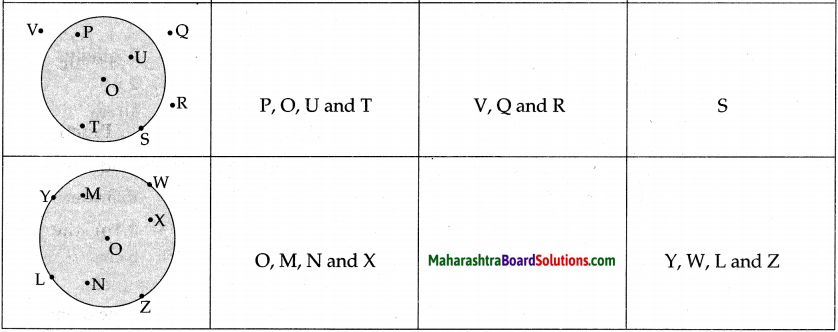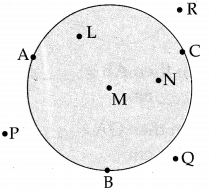# Maharashtra Board Class 5 Maths Solutions Chapter 7 Circles Problem Set 30

Balbharti Maharashtra Board Class 5 Maths Solutions Chapter 7 Circles Problem Set 30 Textbook Exercise Important Questions and Answers.

## Maharashtra State Board Class 5 Maths Solutions Chapter 7 Circles Problem Set 30

Question 1.
In the table below, write the names of the points in the interior and exterior of the circle and those on the circle.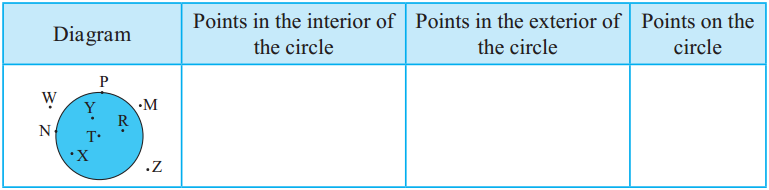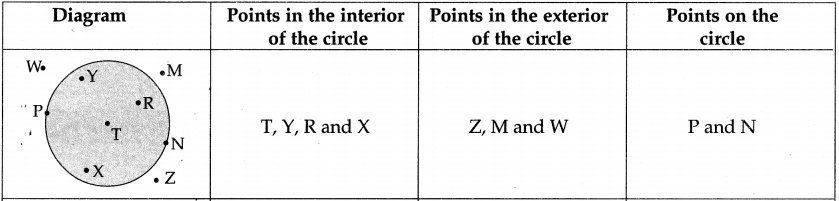The circumference of a circle

Take a bowl with a circular edge.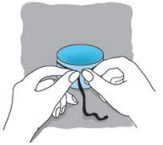Wind a string once around the bowl and make a full circle around it.
Unwind this circle and straighten it out as shown.
Measure the straightened part with a ruler. The length of that part is the circumference of the circle or of the bowl.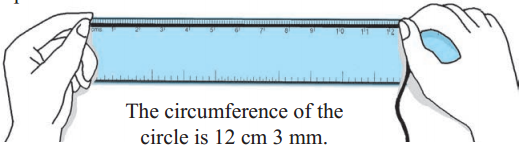An arc of a circle
Shown alongside is a plastic bangle. If the bangle breaks at points A and B, it will split into two parts as shown in the picture.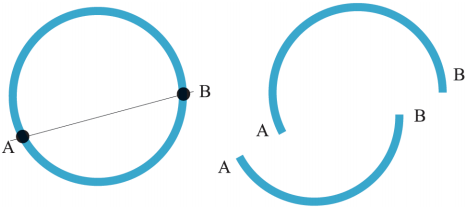Each of these parts is an arc of a circle.On the given circle, there are two points P and Q. These two points have divided the circle into two parts. Each of these parts is an arc of the circle.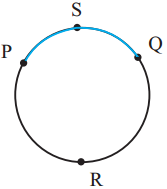This means that P and Q have created two arcs. P and Q are the end points of both arcs.

From the name ‘arc PQ’, we cannot say which of the two arcs we are speaking of. So, an additional point is taken on each arc. This point is used to give each arc a three-letter name. In the figure, there are two arcs, arc PSQ and arc PRQ.